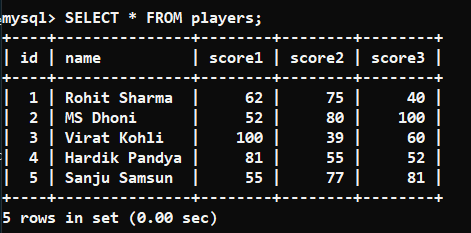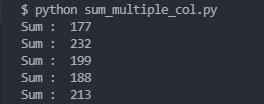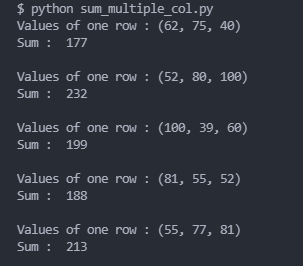GFG App
Open AppBrowser
Continue

In this article, we are going to sum multiple columns and get results using the SQLAlchemy module of python.

## Installation

To install the SQLAlchemy module, run the following command in your terminal:

`pip install sqlalchemy pymysql`

Note: pymysql is a dependency of SQLAlchemy which we need to install for this post.

So, what we are going to do is, firstly we will connect with the database using the SQLAlchemy module, and then using our SQL and python skills we need to sum values of multiple columns and get results.

Database used:So, for this post, we will find out the total score made by all players. And we can do that by adding up “score1”, “score2” and “score3” columns of the player’s table.

Method 1: Firstly, we need to create a connection with our database using SQLAlchemy. Then we will execute a “SELECT” query on the table and inside it we will add the columns. And finally, we will fetch the result.

The SQL query will look like this:

`SELECT column1 + column2 + .... + columnN  FROM table_name;`

You can add any no. of columns you want. Just specify them in the above manner.

The python code will look like this:

## Python3

 `from` `sqlalchemy ``import` `create_engine ` ` `  `user, password, host, database ``=` `'root'``, ``'123'``, ``'localhost'``, ``'geeksforgeeks'` `engine ``=` `create_engine( ` `    ``url``=``f``'mysql+pymysql://{user}:{password}@{host}/{database}?charset=utf8'``) ` ` `  `connection ``=` `engine.connect() ` ` `  `table_name ``=` `'players'` `column1 ``=` `'score1'` `column2 ``=` `'score2'` `column3 ``=` `'score3'` ` `  `result ``=` `connection.execute( ` `    ``f``'SELECT {column1} + {column2} + {column3} FROM {table_name}'``) ` ` `  `for` `value ``in` `result: ` `      ``sum_value ``=` `value[``0``] ` `    ``print``(``"Sum : "``,sum_value) `Method 2: Another way of doing the same task is by running a normal “SELECT” query and mentioning all the columns which we want to add inside the query. Then we will fetch the result and run a for loop on it. The element of the result will be a tuple that contains the values of all the columns of a single row, and we will add them up to get the total of them.

The SQL query will look like this:

`SELECT column1 , column2 , .... , columnN FROM table_name;`

Example:

## Python3

 `from` `sqlalchemy ``import` `create_engine ` ` `  `user, password, host, database ``=` `'root'``, ``'123'``, ``'localhost'``, ``'geeksforgeeks'` `engine ``=` `create_engine( ` `    ``url``=``f``'mysql+pymysql://{user}:{password}@{host}/{database}?charset=utf8'``) ` ` `  `connection ``=` `engine.connect() ` ` `  `table_name ``=` `'players'` ` `  `column1 ``=` `'score1'` `column2 ``=` `'score2'` `column3 ``=` `'score3'` ` `  `result ``=` `connection.execute( ` `    ``f``'SELECT {column1} , {column2} , {column3} FROM {table_name}'``) ` ` `  `for` `value ``in` `result: ` `    ``print``(``"Values of one row :"``, value) ` `    ``sum_value ``=` `sum``(value) ` `    ``print``(``"Sum : "``, sum_value) `My Personal Notes arrow_drop_up